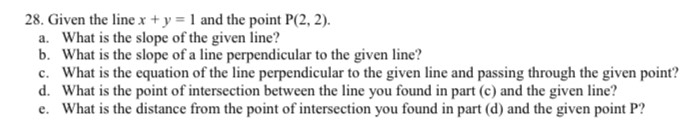### ¿Todavía tienes preguntas de matemáticas?

Pregunte a nuestros tutores expertos
Algebra
Pregunta28. Given the line $$x + y = 1$$ and the point $$P ( 2,2 )$$ . a. What is the slope of the given line? b. What is the slope of a line perpendicular to the given line? c. What is the equation of the line perpendicular to the given line and passing through the given point? d. What is the point of intersection between the line you found in part (c) and the given line? e. What is the distance from the point of intersection you found in part (d) and the given point $$P$$ ?

$$y= x$$
$$(\frac{1}{2} ,\frac{1}{2} )$$
$$\frac { 3 } { 2 } \sqrt { 2 }$$